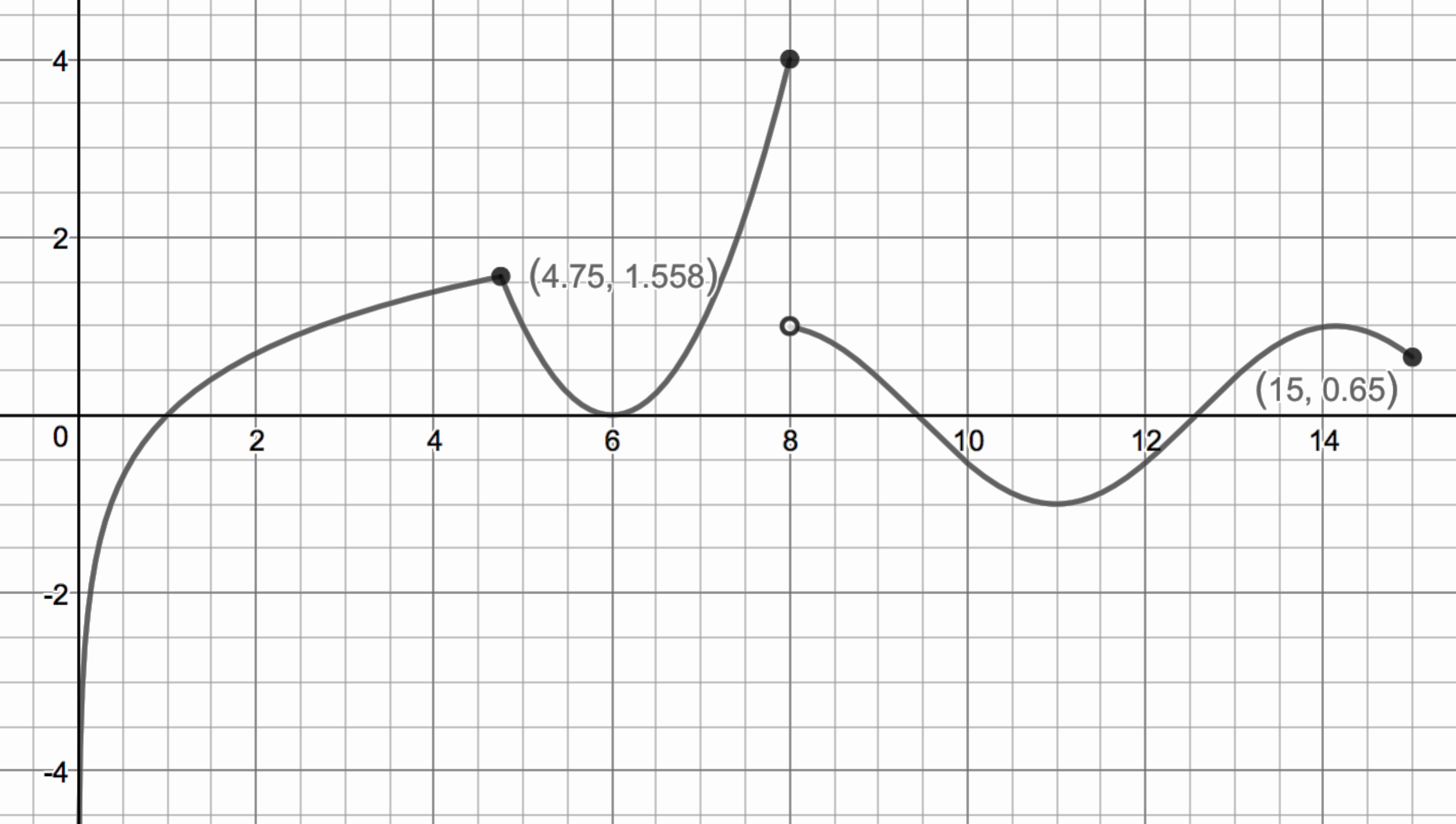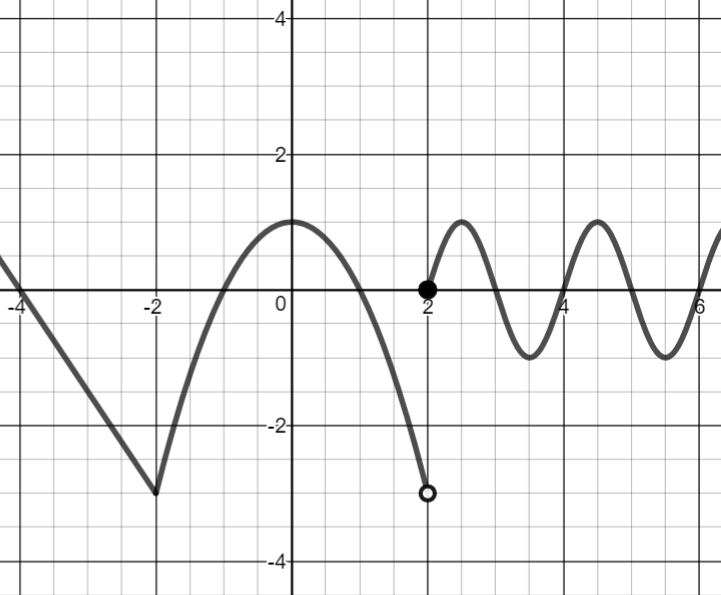# Limits and Continuity

## Objective

Write and evaluate piecewise functions algebraically and graphically using parent functions.

## Criteria for Success

?

1. Know the structure and domain of 12 parent functions.
2. Match domains of a piecewise graph with an appropriate parent function.
3. Write piecewise functions using transformations of parent functions.
4. Evaluate limits of nonlinear piecewise functions.
5. Describe continuity of nonlinear piecewise functions.

## Tips for Teachers

?

This lesson is aligned to the Learning Objectives and Essential Knowledge described in the College Board's AP Calculus AB and AP Calculus BC Course and Exam Description:

LO1.1A(b): EK1.1A1, EK1.1A2, EK1.1A3

LO1.1B: EK1.1B1

LO1.2A: EK1.2A1, EK1.2A2 (approaching)

## Anchor Problems

?

### Problem 1

Draw a sketch of each of the parent functions shown in the table below.

Parent functions:

 ${\mathrm{ln}(x)}$ ${e^x}$ ${x^2}$ ${x^3}$ ${|x|}$ $c$ (where $c$ is a constant) ${\sqrt{x}}$ ${\sqrt{x}}$ ${\mathrm{sin}(x)}$ $\mathrm{cos}(x)$ ${1\over x}$ $x$

### Problem 2

Write the piecewise function for the graph shown below.## Problem Set

?

The following resources include problems and activities aligned to the objective of the lesson that can be used to create your own problem set.

• Given the graph of a piecewise function, write the function algebraically with domain restrictions. Don’t need to do any more than two pieces
• Given the equations of a piecewise function, draw the graph. Don’t need to do any more than two pieces
• Practice other parts of the unit as necessary within the questions of the problem set
• Adapt questions to focus on parent functions that students need additional practice with
• Emphasize interval notation on infinite domains (for instance, ${(3, \infty)}$) in this lesson